Next: 1.6 Spike Codes Up: 1. Introduction Previous: 1.4 The Problem of

Subsections

# 1.5 Rate Codes

A quick glance at the experimental literature reveals that there is no unique and well-defined concept of mean firing rate'. In fact, there are at least three different notions of rate which are often confused and used simultaneously. The three definitions refer to three different averaging procedures: either an average over time, or an average over several repetitions of the experiment, or an average over a population of neurons. The following three subsections will reconsider the three concepts. An excellent discussion of rate codes can be found in (Rieke et al., 1996).

## 1.5.1 Rate as a Spike Count (Average over Time)

The first and most commonly used definition of a firing rate refers to a temporal average. As discussed in the preceding section, this is essentially the spike count in an interval of duration T divided by T; see Fig. 1.9. The length T of the time window is set by the experimenter and depends on the type of neuron recorded from and the stimulus. In practice, to get sensible averages, several spikes should occur within the time window. Typical values are T = 100 ms or T = 500 ms, but the duration may also be longer or shorter.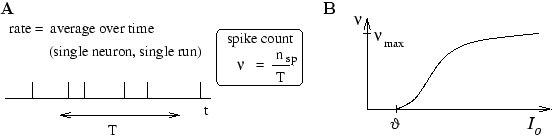This definition of rate has been successfully used in many preparations, particularly in experiments on sensory or motor systems. A classical example is the stretch receptor in a muscle spindle (Adrian, 1926). The number of spikes emitted by the receptor neuron increases with the force applied to the muscle. Another textbook example is the touch receptor in the leech (Kandel and Schwartz, 1991). The stronger the touch stimulus, the more spikes occur during a stimulation period of 500 ms.

These classical results show that the experimenter as an external observer can evaluate and classify neuronal firing by a spike count measure - but is this really the code used by neurons in the brain? In other words, is a neuron which receives signals from a sensory neuron only looking at and reacting to the number of spikes it receives in a time window of, say, 500 ms? We will approach this question from a modeling point of view later on in the book. Here we discuss some critical experimental evidence.

From behavioral experiments it is known that reaction times are often rather short. A fly can react to new stimuli and change the direction of flight within 30-40 ms; see the discussion in (Rieke et al., 1996). This is not long enough for counting spikes and averaging over some long time window. The fly has to respond after a postsynaptic neuron has received one or two spikes. Humans can recognize visual scenes in just a few hundred milliseconds (Thorpe et al., 1996), even though recognition is believed to involve several processing steps. Again, this does not leave enough time to perform temporal averages on each level. In fact, humans can detect images in a sequence of unrelated pictures even if each image is shown for only 14 - 100 milliseconds (Keysers et al., 2001).

Temporal averaging can work well in cases where the stimulus is constant or slowly varying and does not require a fast reaction of the organism - and this is the situation usually encountered in experimental protocols. Real-world input, however, is hardly stationary, but often changing on a fast time scale. For example, even when viewing a static image, humans perform saccades, rapid changes of the direction of gaze. The image projected onto the retinal photo receptors changes therefore every few hundred milliseconds.

Despite its shortcomings, the concept of a firing rate code is widely used not only in experiments, but also in models of neural networks. It has led to the idea that a neuron transforms information about a single input variable (the stimulus strength) into a single continuous output variable (the firing rate); cf. Fig. 1.9B. The output rate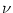increases with the stimulus strength and saturates for large input I0 towards a maximum value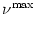. In experiments, a single neuron can be stimulated by injecting with an intra-cellular electrode a constant current I0. The relation between the measured firing frequencyand the applied input current I0 is sometimes called the frequency-current curve of the neuron. In models, we formalize the relation between firing frequency (rate) and input current and write= g(I0). We refer to g as the neuronal gain function or transfer function.

From the point of view of rate coding, spikes are just a convenient way to transmit the analog output variableover long distances. In fact, the best coding scheme to transmit the value of the ratewould be by a regular spike train with intervals 1/. In this case, the rate could be reliably measured after only two spikes. From the point of view of rate coding, the irregularities encountered in real spike trains of neurons in the cortex must therefore be considered as noise. In order to get rid of the noise and arrive at a reliable estimate of the rate, the experimenter (or the postsynaptic neuron) has to average over a larger number of spikes. A critical discussion of the temporal averaging concept can be found in (Shadlen and Newsome, 1994; Softky, 1995; Rieke et al., 1996).

## 1.5.2 Rate as a Spike Density (Average over Several Runs)

There is a second definition of rate which works for stationary as well as for time-dependent stimuli. The experimenter records from a neuron while stimulating with some input sequence. The same stimulation sequence is repeated several times and the neuronal response is reported in a Peri-Stimulus-Time Histogram (PSTH); see Fig. 1.10. The time t is measured with respect to the start of the stimulation sequence and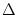t is typically in the range of one or a few milliseconds. The number of occurrences of spikes nK(t;t +t) summed over all repetitions of the experiment divided by the number K of repetitions is a measure of the typical activity of the neuron between time t and t +t. A further division by the interval lengtht yields the spike density of the PSTH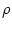(t) =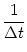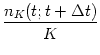. (1.9)

Sometimes the result is smoothed to get a continuous rate' variable. The spike density of the PSTH is usually reported in units of Hz and often called the (time-dependent) firing rate of the neuron.As an experimental procedure, the spike density measure is a useful method to evaluate neuronal activity, in particular in the case of time-dependent stimuli. The obvious problem with this approach is that it can not be the decoding scheme used by neurons in the brain. Consider for example a frog which wants to catch a fly. It can not wait for the insect to fly repeatedly along exactly the same trajectory. The frog has to base its decision on a single run' - each fly and each trajectory is different.

Nevertheless, the experimental spike density measure can make sense, if there are large populations of independent neurons that receive the same stimulus. Instead of recording from a population of N neurons in a single run, it is experimentally easier to record from a single neuron and average over N repeated runs. Thus, the spike density coding relies on the implicit assumption that there are always populations of neurons and therefore leads us to the third notion of a firing rate, viz., a rate defined as a population average.

## 1.5.3 Rate as a Population Activity (Average over Several Neurons)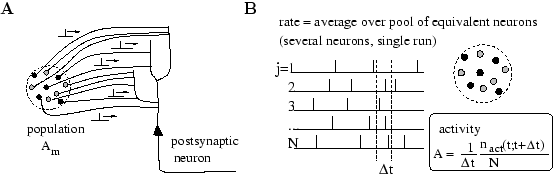The number of neurons in the brain is huge. Often many neurons have similar properties and respond to the same stimuli. For example, neurons in the primary visual cortex of cats and monkeys are arranged in columns of cells with similar properties (Hubel, 1988; Hubel and Wiesel, 1962,1977). Let us idealize the situation and consider a population of neurons with identical properties. In particular, all neurons in the population should have the same pattern of input and output connections. The spikes of the neurons in a population m are sent off to another population n. In our idealized picture, each neuron in population n receives input from all neurons in population m. The relevant quantity, from the point of view of the receiving neuron, is the proportion of active neurons in the presynaptic population m; see Fig. 1.11A. Formally, we define the population activity

 A(t) ==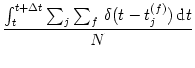(1.10)

where N is the size of the population, nact(t;t +t) the number of spikes (summed over all neurons in the population) that occur between t and t +t andt a small time interval; see Fig. 1.11. Eq. (1.10) defines a variable with units s-1 - in other words, a rate.

The population activity may vary rapidly and can reflect changes in the stimulus conditions nearly instantaneously (Brunel et al., 2001; Gerstner, 2000a). Thus the population activity does not suffer from the disadvantages of a firing rate defined by temporal averaging at the single-unit level. A potential problem with the definition (1.10) is that we have formally required a homogeneous population of neurons with identical connections which is hardly realistic. Real populations will always have a certain degree of heterogeneity both in their internal parameters and in their connectivity pattern. Nevertheless, rate as a population activity (of suitably defined pools of neurons) may be a useful coding principle in many areas of the brain. For inhomogeneous populations, the definition (1.10) may be replaced by a weighted average over the population.

### 1.5.3.1 Example: Population vector coding

We give an example of a weighted average in an inhomogeneous population. Let us suppose that we are studying a population of neurons which respond to a stimulus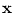. We may think ofas the location of the stimulus in input space. Neuron i responds best to stimulus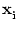, another neuron j responds best to stimulus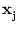. In other words, we may say that the spikes for a neuron i represent' an input vectorand those of j an input vector. In a large population, many neurons will be active simultaneously when a new stimulusis represented. The location of this stimulus can then be estimated from the weighted population average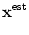(t) =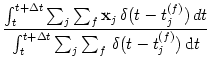(1.11)

Both numerator and denominator are closely related to the population activity (1.10). The estimate (1.11) has been successfully used for an interpretation of neuronal activity in primate motor cortex (Wilson and McNaughton, 1993; Georgopoulos et al., 1986). It is, however, not completely clear whether postsynaptic neurons really evaluate the fraction (1.11). In any case, eq. (1.11) can be applied by external observers to `decode' neuronal signals, if the spike trains of a large number of neurons are accessible.Next: 1.6 Spike Codes Up: 1. Introduction Previous: 1.4 The Problem of
Gerstner and Kistler
Spiking Neuron Models. Single Neurons, Populations, Plasticity
Cambridge University Press, 2002

© Cambridge University Press
This book is in copyright. No reproduction of any part of it may take place without the written permission of Cambridge University Press.